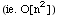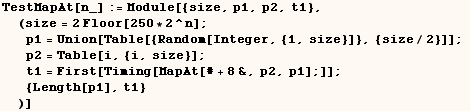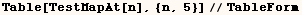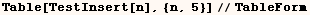Slow Kernel Functions

Functions ReplacePart, MapAt, Insert, AppendTo, and PrependTo are very  slowwhen they are used to make a large number of changes to an expression.   The other functions I list here are not extremly slow, but they are all much  slower than the simpler form of the same function (eg. Sort[list,p] is much  slower than Sort[list]).

ReplacePart, MapAt, Insert (when the last argument is a long list).

ReplacePart, MapAt, and Inset can be very slow.

Consider the expressions:

ReplacePart[expr, 1, lst]

MapAt[f,expr, lst]

Inset[expr, 1, lst]

Where (lst) has the form {{__Integer},{__Integer}, ...] and Length[lst]=n.

In that case each of these functions are O[n^2].  For those who aren't
familiar with this notation, O[n^2] means the evaluation time is proportional
to n^2.  Hence if (lst) is a long list of lists these functions are very
slow.

Here is the evidence395 0.05 Second 780 0.22 Second 1580 0.93 Second 3108 4.01 Second 6276 18.12 Second396 0.06 Second 782 0.27 Second 1593 1.26 Second 3191 5.49 Second 6272 24.28 Second237 0.06 Second 472 0.11 Second 951 0.49 Second 1879 1.98 Second 3786 8.4 Second

Would a faster algorithm give "wrong" results?

It seems like there must be algorithms for (ReplacePart, MapAt, Insert) that
would evaluate much faster with a long list of parts.  It may be that the
developers used slower methods which are needed to ensure that the result is
correct.  A similar issue had to be addressed with Union.  However, in the
case of Union the developers decided to use a method that is O[n Log[n]] and
they accepted the risk that the result might include some duplicates.

At  http://support.wolfram.com/Kernel/Symbols/System/Union.html  we see Union treats elements as duplicates only if they appear in  adjacent positions after sorting.  Apparently this method is O[n Log[n]], and  Union would be O[n^2] if it compared all pairs of elements.

AppendTo, PrependTo

AppendTo and PrependTo are very slow when used on large expressions.   Instead use a "linked list" as I discuss under programming for speed.

Sort[list,p]

Sort[list]  is faster than  Sort[list, p] but sometimes we can't avoid the
slower form.

Ordering[list,n,p]

Ordering[list, n, p] is slower than  Ordering[list]  or Ordering[list, n] but
sometimes we can't avoid the slower form.

Split[list,test]

Split[list, test]  is slower than  Split[list] but sometimes we can't avoid
the slower form.

MatrixQ[expr,test], VectorQ[expr,test]

MatrixQ[expr, test]  is slower than  MatrixQ[expr].

Likewise  VectorQ[expr, test]  is slower than  VectorQ[expr].

However, sometimes we can't avoid the slower form of VectorQ and MatrixQ.

(SameTest→func)  instead of (SameTest→Automatic)

The functions (Complement, FixedPoint, FixedPointList, Intersection, Union)
all have a SameTest option. When they are given a non-default setting for the
SameTest option they run much slower that with the default setting (SameTest
→Automatic).  Sometimes we have no choice but to use these functions
with a non default SameTest setting.  You should just be aware that this
slows down performance.

Created by Mathematica  (May 17, 2004)